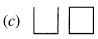# PSEB 3rd Class Maths Solutions Chapter 7 Patterns

Punjab State Board PSEB 3rd Class Maths Book Solutions Chapter 7 Patterns Textbook Exercise Questions and Answers.

## PSEB Solutions for Class 3 Maths Chapter 7 Patterns

PAGE 152:

Do you remember?

See carefully and fill in the blanks :Solution.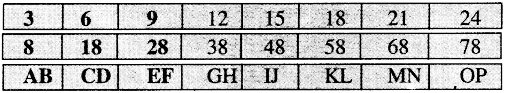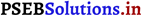PAGE 154:
Let’s do:

Observe the patterns and complete them: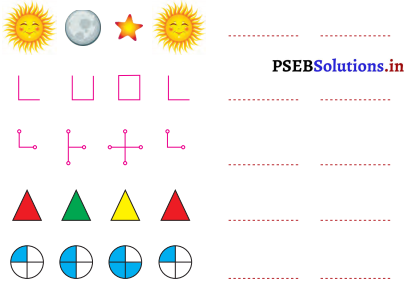Solution.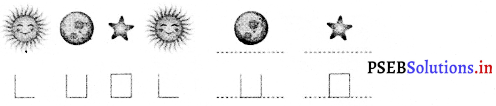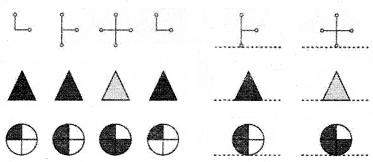Make some beautiful patterns with your thumb:Solution.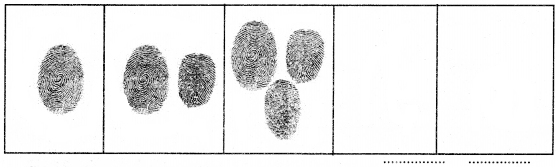As first pattern is given below, mark the next pattern:Solution.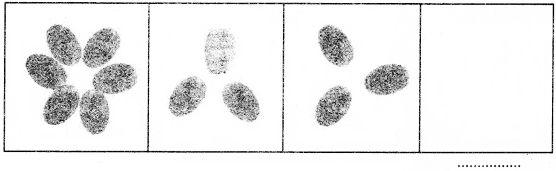PAGE 157:
Let’s do:

Question 1.
Observe the patterns and complete the following: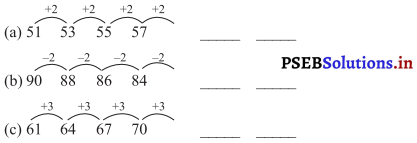Solution.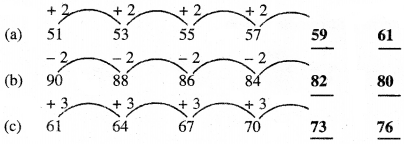Question 2.
Add the odd numbers and complete the following patterns:
Solution.
(a) 1 + 3 + 5 + 7 + 9 + 11 = 6 × 6

(b) 1 + 3 + 5 + 7 + 9 + 11 + 13 = 7 × 7

(c) 1 + 3 + 5 + 7 + 9 + 11 + 13 + 15 = _____
Solution.
8 × 8

(d) 1 + 3 + 5 + 7 + 9 + 11 + 13 + 15 + 17 = _____
Solution.
9 × 9

Question 3.
Add the even numbers and complete the following patterns :
(a) 2 + 4 + 6 + 8 + 10 = 5 × 6

(b) 2 + 4 + 6 + 8 + 10 + 12 = 6 × 7

(c) 2 + 4 + 6 + 8 + 10 + 12 + 14 = _____
Solution.
7 × 8

(d) 2 + 4 + 6 + 8 + 10 + 12 + 14 + 16 = _____
Solution.
8 × 9Question 4.
Observe the pattern carefully and complete it: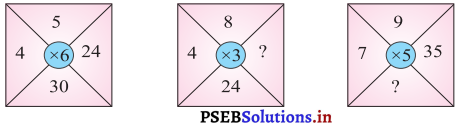Solution.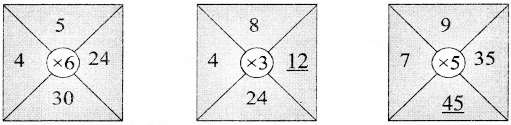Multiple Choice Questions:

Question 1.
Complete the pattern: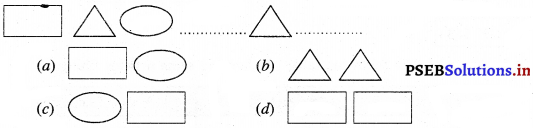Solution.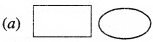Question 2.
Naman has proud of his particular name. He says that if his name is read inversely it will remain the same. Find this pattern in the following names? Harsh, Daksh, Amar, Kanak, Aman.
(a) Harsh
(b) Daksh
(c) Amar
(d) Kanak.
Solution.
(d) Kanak.

Question 3.
Complete the Pattern :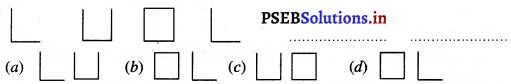Solution.